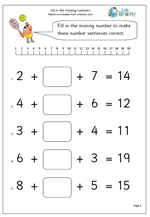# Y2 addition worksheet: find the missing numberSometimes sums can prove to be more difficult than they first appear. For many children this is the case with the kind of questions on this worksheet which require finding the missing number. Why is this difficult? Because it involves carrying out two processes, when children are used to only having to do one thing to find the answer. Whilst it is an addition number sentence, subtraction is needed to work out the answer.

There are several methods which could be used to find the answer, but the most efficient is probably to:

1. Add the two single digits

2. Subtract that total from the answer.

Another way is to start with the answer and find it on the number line, then hop back the first number, then hop back the second number. Where you land is the answer.

Find the missing number: adding 3 numbers